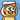October 05, 2018

# 分析

• 在 JavaScript 中，`Number`的取值范围从最小`-(2^53 - 1)`到最大`(2^53 - 1)` (参考 MDN)。如果变量的值超过这两个界限的话就会变成`-Infinite``Infinite`。因此，假如我们要做千位数以上的乘法，就不能使用`Number`作为变量类型，而只能使用`String`。同样的，返回的结果也应该是`String`才能避免溢出。
• 在计算过程中，需要应用分治思想，一个数字一个数字地乘上去，然后再考虑数字进位的问题。
• 将最终得到的结果转换成为`String`的格式输出

# 实际操作

## 第一步 定义输入与输出

```/** * Multiplication of two big numbers * @param {String} num1 big number which may exceed javascript native number limitation * @param {String} num2 big number which may exceed javascript native number limitation * @returns {String} The product of the two big numbers */ function bigNumberMultiplication(num1, num2) { // Implementation }```

## 第二步 使用数组储存每个数字

1. 使用`toString()`将变量转换为`String`
2. 使用`split()`将字符串变量的每一个字符分开并生成一个字符数组
3. 使用`map()`将字符数组里面的所有字符通过`parseInt()`方法转换成`Number`
4. 使用`reverse()`将数组的顺序倒转过来，便于计算，因为数组的第一位是最大位数，而计算是从最小位数开始的

```// reverse the array to start calculation from the smallest digit const a = num1 .toString() .split("") .map(x => parseInt(x)) .reverse(); const b = num2 .toString() .split("") .map(x => parseInt(x)) .reverse();```

## 第三步 储存相乘的积

```const result = []; // iterate every digit and construct an array to record every digit b.forEach((vb, j) => { a.forEach((va, i) => { result[i + j] = (result[i + j] ? result[i + j] : 0) + va * vb; }); });```

## 第四步 处理进位

`result`数组里，每一位数都不一定是个位数。所以这个时候我们就得处理进位的问题。进位问题很容易解决。对每一位数，只要它大于等于 10，就将它缩小 10 倍，然后将它的下一位加 1。直到这一位数处理完毕之后，再对下一位数进行处理。比如：

`[0, 1, 9, 19, 35]` 处理第一位数 0，0 <= 10，去下一位

`[0, 1, 9, 19, 35]` 处理第二位数 1，1 <= 10，去下一位

`[0, 1, 9, 19, 35]` 处理第三位数 1，9 <= 10，去下一位

`[0, 1, 9, 19, 35]` 处理第四位数 19，19 > 10，更新第四位数位`19 % 10`，第五位数加`19 / 10`

`[0, 1, 9, 9, 36]` 处理第四位数 9，9 <= 10，去下一位

`[0, 1, 9, 9, 36]` 处理第五位数 36，19 > 10，更新第五位数位`36 % 10`，第六位数加`36 / 10`

`[0, 1, 9, 9, 6, 3]` 处理第五位数 6，6 <= 10，去下一位

`[0, 1, 9, 9, 6, 3]` 处理第六位数 3，3 <= 10，完成

```// adding the extra values to next digit until every digit is reduced to unit digit let currDigit = 0; let nextDigit; while (result[currDigit] !== undefined) { if (result[currDigit] >= 10) { nextDigit = currDigit + 1; result[nextDigit] = (result[nextDigit] ? result[nextDigit] : 0) + Math.floor(result[currDigit] / 10); result[currDigit] = result[currDigit] % 10; } currDigit++; }```

## 第五步 返回字符串

`return result.reverse().join("");`

# 大功告成！

```/** * Multiplication of two big numbers * @param {String} num1 big number which may exceed javascript native number limitation * @param {String} num2 big number which may exceed javascript native number limitation * @returns {String} The product of the two big numbers */ function bigNumberMultiplication(num1, num2) { // reverse the array to start calculation from the smallest digit const a = num1 .toString() .split("") .map(x => parseInt(x)) .reverse(); const b = num2 .toString() .split("") .map(x => parseInt(x)) .reverse(); const result = []; // iterate every digit and construct an array to record every digit b.forEach((vb, j) => { a.forEach((va, i) => { result[i + j] = (result[i + j] ? result[i + j] : 0) + va * vb; }); }); // adding the extra values to next digit until every digit is reduced to unit digit let currDigit = 0; let nextDigit; while (result[currDigit] !== undefined) { if (result[currDigit] >= 10) { nextDigit = currDigit + 1; result[nextDigit] = (result[nextDigit] ? result[nextDigit] : 0) + Math.floor(result[currDigit] / 10); result[currDigit] = result[currDigit] % 10; } currDigit++; } return result.reverse().join(""); }```Written by Yi Zhiyue
A Software Engineer · 山不在高，有仙则灵